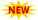## 23 September 2013

### MA2211 / MA31 / 10177 MA301 / 80100008 / MA1201A - Transforms and Partial Differential Equations - Formula Collections by Hariganesh

Anna university

Third Semester

MA2211 / MA31 / 10177 MA301 / 80100008 / MA1201A
Transforms and Partial Differential Equations

Regulation- 2008

Formula Material

Semester: 3

Year: 2

Subject code:  MA2211 / MA31 / 10177 MA301 / 80100008 / MA1201A

Subject Title: Transforms and Partial Differential Equations

Type: Formula Material

Number of Pages: 3

Attachment format : Pdf

Version: Typed Version

Regulation: 2008

Content: 3rd semester  - MA2211 / MA31 / 10177 MA301 / 80100008 / MA1201A - Transforms and partial differential equations formula material.

Prepared by: C.Ganesan, M.Sc., M.phil.,

View 3rd semester  MA2211 / MA31 / 10177 MA301 / 80100008 / MA1201A Transforms and partial differential equations formula material before download

Download a copy of 3rd semester  MA2211 / MA31 / 10177 MA301 / 80100008 / MA1201A Transforms and partial differential equations formula material
Search Terms:
TPDE Notes, Transforms and partial differential equations Notes, Anna University 3rd semster TPDE,
Anna University 3rd semster Transforms and partial differential equations , TPDE Formula Material,
Transforms and partial differential equations formula material, TPDE important Questions,Transforms and Partial Differential Equation question papers and question bank,Transforms and Partial Differential Equation previous year question papers,Tpde question papers,Transforms and Partial Differential Equation question papers,Transforms and Partial Differential Equation, Tpde notes, Maths 3 question papers, maths 3 notes,
M3 notes, M3 question papers, TPDE,  TPDE Formula,  M3 formula collection,  MA2211 Formula materials, MA2211 important formula,  TPDE important formula,  M3 important formulaNeed more material ? Visit www.csetube.in